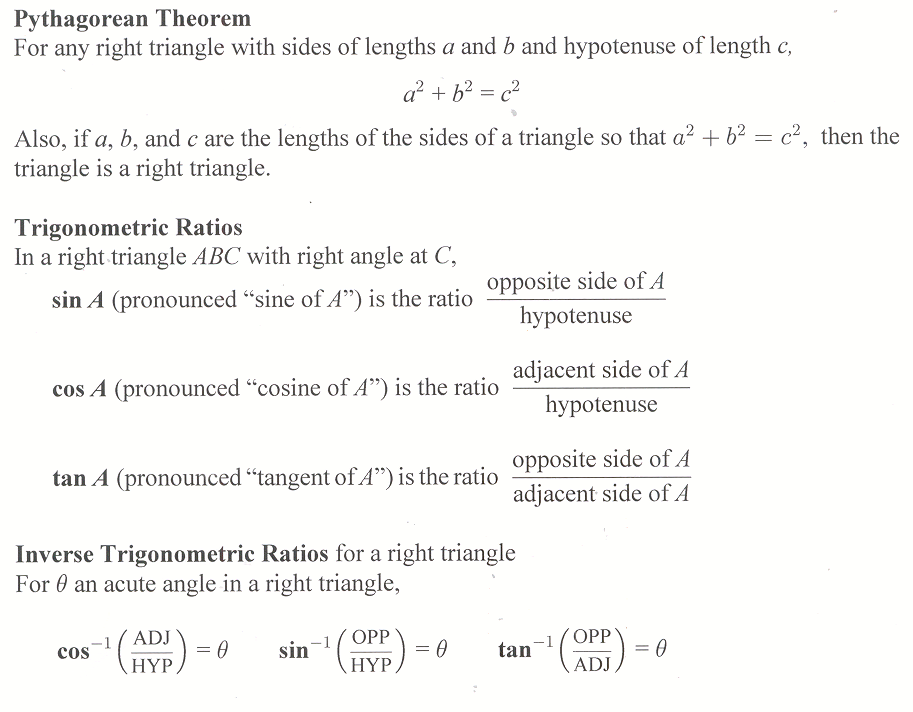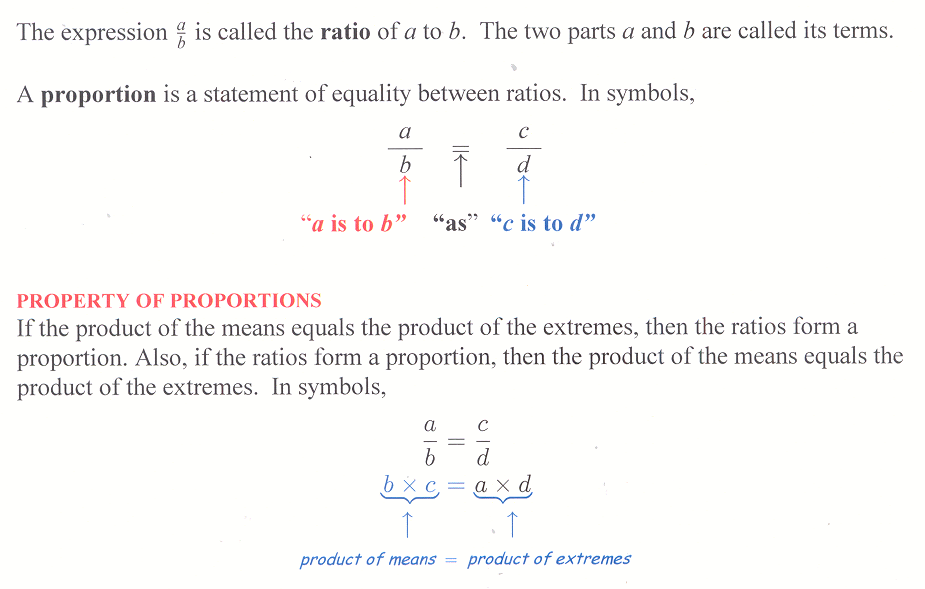## Individual Research Projects Section 7.6

### Project 7.6

Do some research on the length-to-width ratios of the packaging of common household items. Form some conclusions. Find some examples of the golden ratio in art. Do some research on dynamic symmetry.
References:
Philip J. Davis and Reuben See the whole entry

## Homework Hints 7-5

[tippy title=”Problems 1-4″]Problems 1-4
These problems are designed to make sure you are familiar with the Pythagorean theorem as well as the definitions of sine, cosine, and tangent.[/tippy]

[tippy title=”Problem 5″]Problem 5
Use the Pythagorean theorem and Example 1.[/tippy]

[tippy See the whole entry

## 7.5 Outline

1. Pythagorean theorem
2. Trigonometric ratios
1. sine
2. cosine
3. tangent
3. Inverse trigonometric ratios
1. calculator calculating
2. inverse trigonometric ratios
1. inverse tangent
2. inverse cosine
3. inverse sine
4. Applications
1. angle of elevation
2. angle of depression

## 7.5 Essential IdeasSee the whole entry

## 6.7 Outline

1. Ratios
1. definition
2. reduce a ratio
2. Proportions
1. definition
2. property of proportions
3. solving proportions
1. cross-product divided by the number opposite the unknown
2. procedure

## 6.7 Essential IdeasSee the whole entry